Data Visualization

### Exploratory Data Visualization in R¶

In :
WHO <- read.csv("Data/WHO.csv")

In :
str(WHO)

'data.frame':	194 obs. of  13 variables:
$Country : Factor w/ 194 levels "Afghanistan",..: 1 2 3 4 5 6 7 8 9 10 ...$ Region                       : Factor w/ 6 levels "Africa","Americas",..: 3 4 1 4 1 2 2 4 6 4 ...
$Population : int 29825 3162 38482 78 20821 89 41087 2969 23050 8464 ...$ Under15                      : num  47.4 21.3 27.4 15.2 47.6 ...
$Over60 : num 3.82 14.93 7.17 22.86 3.84 ...$ FertilityRate                : num  5.4 1.75 2.83 NA 6.1 2.12 2.2 1.74 1.89 1.44 ...
$LifeExpectancy : int 60 74 73 82 51 75 76 71 82 81 ...$ ChildMortality               : num  98.5 16.7 20 3.2 163.5 ...
$CellularSubscribers : num 54.3 96.4 99 75.5 48.4 ...$ LiteracyRate                 : num  NA NA NA NA 70.1 99 97.8 99.6 NA NA ...
$GNI : num 1140 8820 8310 NA 5230 ...$ PrimarySchoolEnrollmentMale  : num  NA NA 98.2 78.4 93.1 91.1 NA NA 96.9 NA ...
$PrimarySchoolEnrollmentFemale: num NA NA 96.4 79.4 78.2 84.5 NA NA 97.5 NA ...  In : library(ggplot2)  In : scatterplot <- ggplot(WHO, aes(x=GNI, y=FertilityRate)) + geom_point(color="darkred", size=2, shape=20) scatterplot + ggtitle("Fertility Rate vs. Gross National Income") + xlab("Gross National Income") + ylab("Fertility Rate")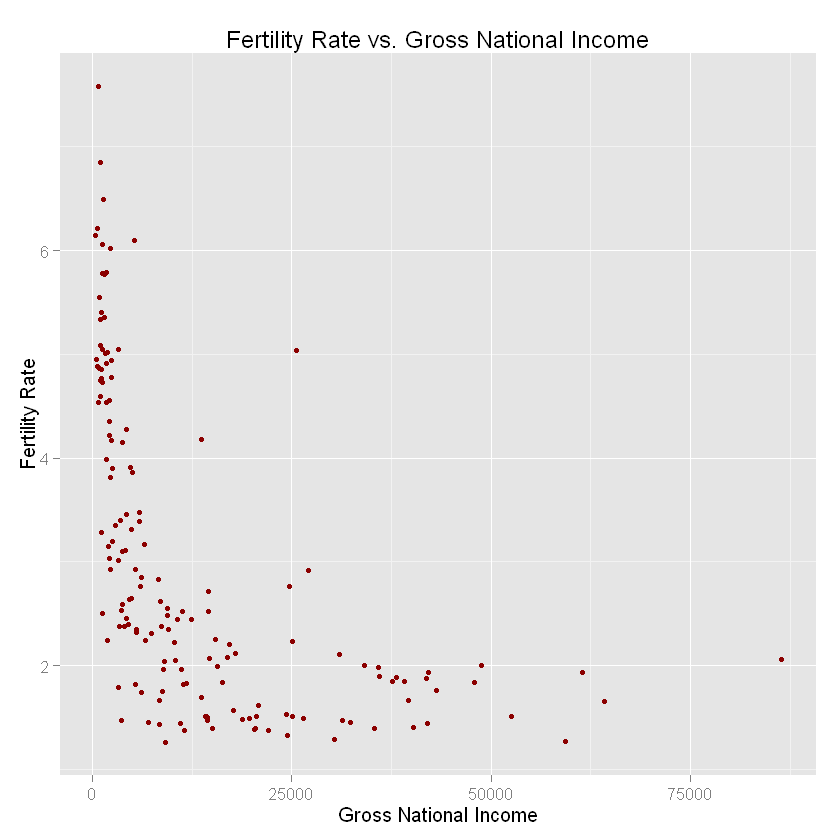In : #save the plot fertilityGNIplot <- scatterplot + ggtitle("Fertility Rate vs. Gross National Income")  In : ggplot(WHO, aes(x=GNI, y=FertilityRate, color=Region)) + geom_point() + xlab("Gross National Income") + ylab("Fertility Rate")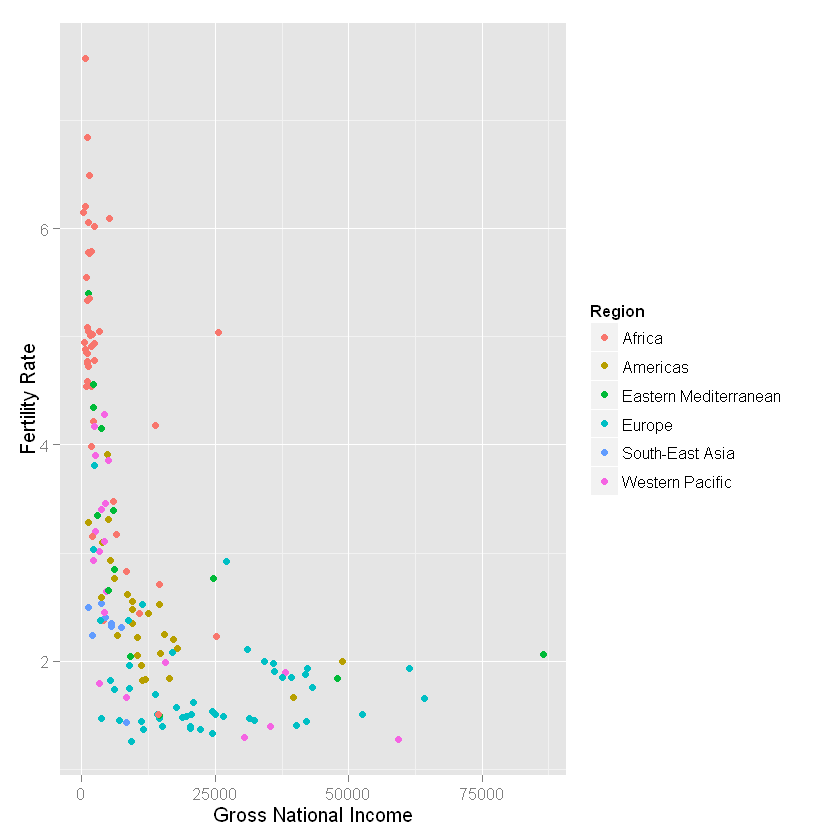In : ggplot(WHO, aes(x=GNI, y=FertilityRate, color=LifeExpectancy)) + geom_point() + xlab("Gross National Income") + ylab("Fertility Rate")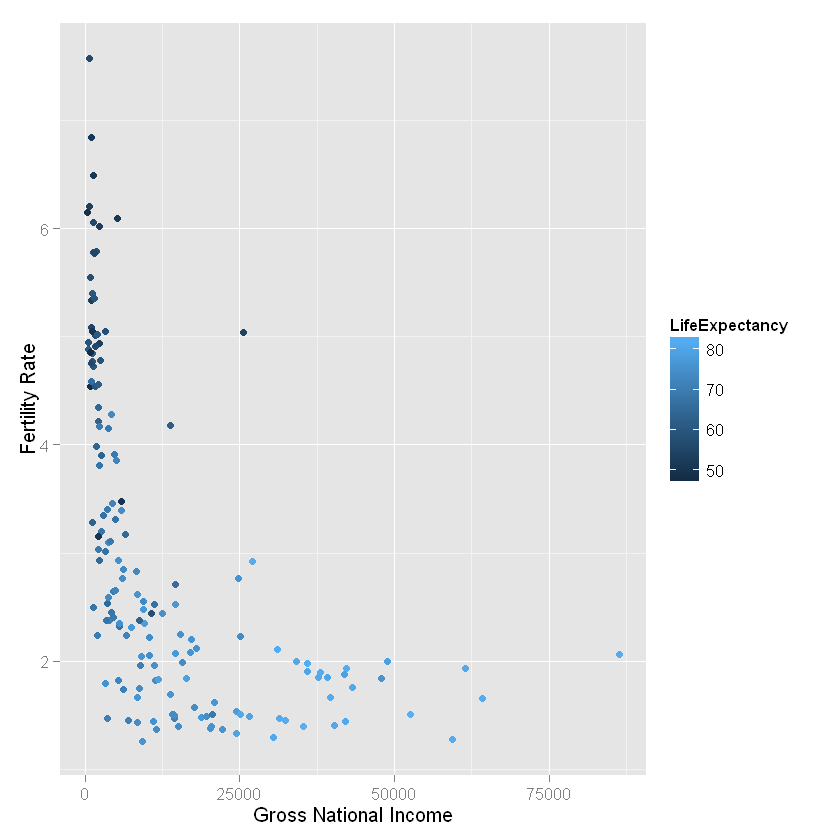In : ggplot(WHO, aes(x=FertilityRate, y=Under15)) + geom_point() + xlab("Fertility Rate")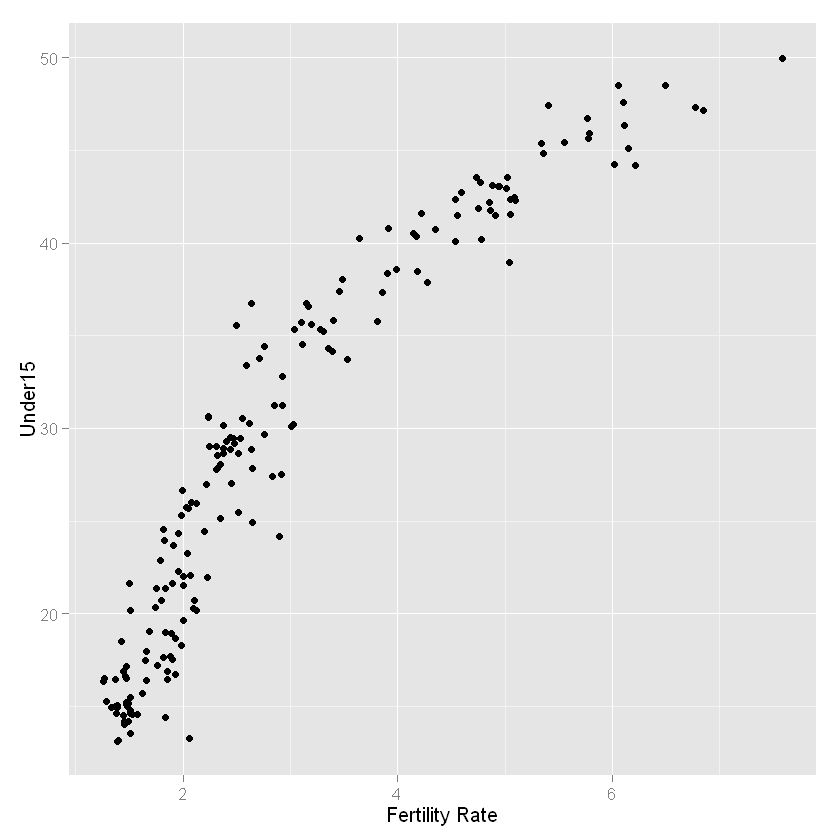In : # transformation seems need here ggplot(WHO, aes(x=log(FertilityRate), y=Under15)) + geom_point()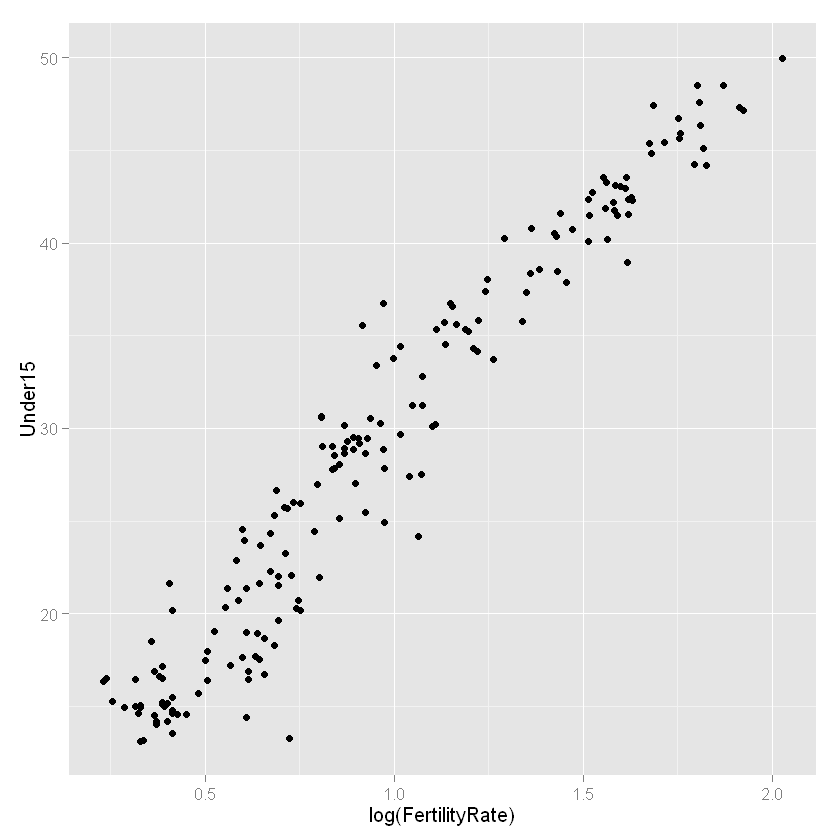The fit seems better now and we can check it by building a linear regression model. In : # a model without transformation summary(lm(Under15 ~ FertilityRate, data=WHO))  Out: Call: lm(formula = Under15 ~ FertilityRate, data = WHO) Residuals: Min 1Q Median 3Q Max -10.3340 -3.0347 0.0219 2.9298 9.8841 Coefficients: Estimate Std. Error t value Pr(>|t|) (Intercept) 9.0161 0.6221 14.49 <2e-16 *** FertilityRate 6.7689 0.1891 35.80 <2e-16 *** --- Signif. codes: 0 '***' 0.001 '**' 0.01 '*' 0.05 '.' 0.1 ' ' 1 Residual standard error: 3.777 on 181 degrees of freedom (11 observations deleted due to missingness) Multiple R-squared: 0.8763, Adjusted R-squared: 0.8756 F-statistic: 1282 on 1 and 181 DF, p-value: < 2.2e-16  In : # a model with transformation summary(lm(Under15 ~ log(FertilityRate), data=WHO))  Out: Call: lm(formula = Under15 ~ log(FertilityRate), data = WHO) Residuals: Min 1Q Median 3Q Max -10.3131 -1.7742 0.0446 1.7440 7.7174 Coefficients: Estimate Std. Error t value Pr(>|t|) (Intercept) 7.6540 0.4478 17.09 <2e-16 *** log(FertilityRate) 22.0547 0.4175 52.82 <2e-16 *** --- Signif. codes: 0 '***' 0.001 '**' 0.01 '*' 0.05 '.' 0.1 ' ' 1 Residual standard error: 2.65 on 181 degrees of freedom (11 observations deleted due to missingness) Multiple R-squared: 0.9391, Adjusted R-squared: 0.9387 F-statistic: 2790 on 1 and 181 DF, p-value: < 2.2e-16  clearly, a model with log(FertilityRate) provides a better fit. Let's now improve the plot by adding a regression line In : # linear model with 95% confidence interval ggplot(WHO, aes(x=log(FertilityRate), y=Under15)) + geom_point(color="darkred", size=2, shape=20) + stat_smooth(method="lm")  Error in +stat_smooth(method = "lm"): invalid argument to unary operator In : # linear model with 99% confidence interval # change the color of the fitted line ggplot(WHO, aes(x=log(FertilityRate), y=Under15)) + geom_point(color="darkred", size=2, shape=20) + stat_smooth(method="lm", level=0.99, color = "darkblue")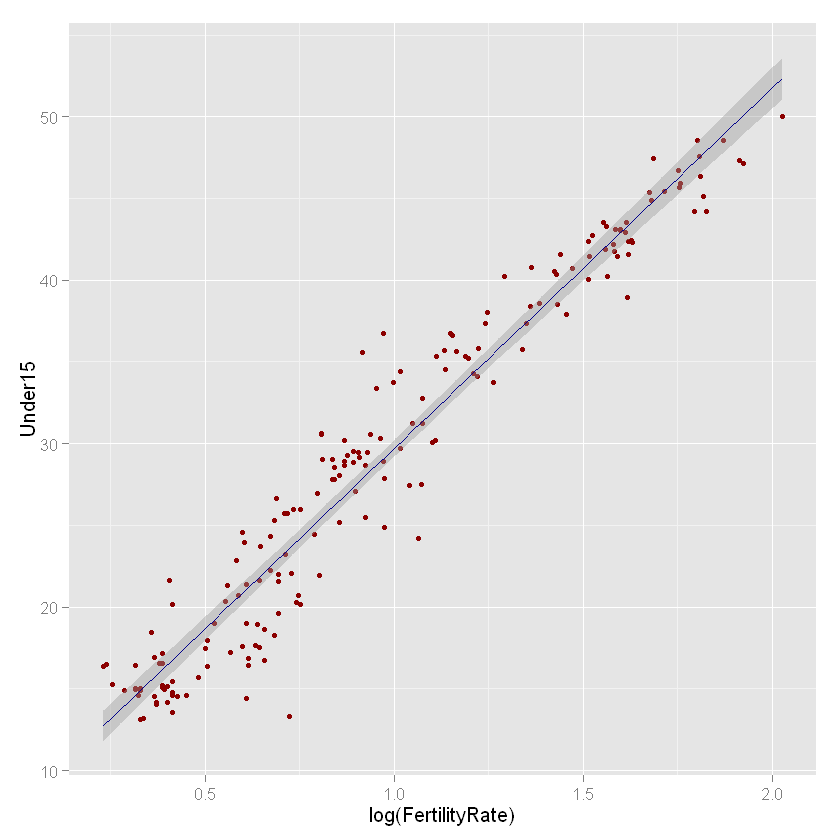In : # remove the confidence interval ggplot(WHO, aes(x=log(FertilityRate), y=Under15)) + geom_point(color="darkred", size=2, shape=20) + stat_smooth(method="lm", se=FALSE, color = "darkblue")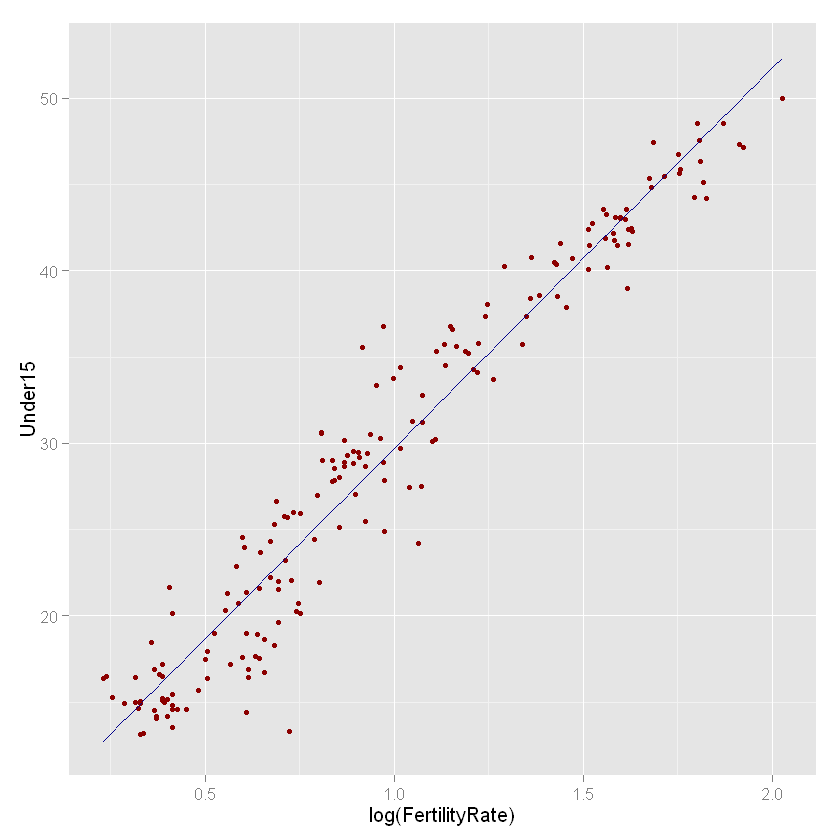### Viusalizing Motor Vehicle Theft¶ This subsection will provide a visual representation of vehicle thefts in a region. In : mvt <- read.csv("Data/mvt.csv", stringsAsFactors=FALSE)  In : head(mvt)  Out: DateLatitudeLongitude 112/31/12 23:1541.75628-87.62164 212/31/12 22:0041.89879-87.6613 312/31/12 22:0041.96919-87.76767 412/31/12 22:0041.76933-87.65773 512/31/12 21:3041.83757-87.62176 612/31/12 20:3041.92856-87.754 First convert the date to a format that is more convinient In : # Convert the Date variable to a format that R will recognize: mvt$Date = strptime(mvt$Date, format="%m/%d/%y %H:%M") # Extract the hour and the day of the week: mvt$Weekday = weekdays(mvt$Date) mvt$Hour = mvt$Date$hour

# Let's take a look at the structure of our data again:
str(mvt)

'data.frame':	191641 obs. of  5 variables:
$Date : POSIXlt, format: "2012-12-31 23:15:00" "2012-12-31 22:00:00" ...$ Latitude : num  41.8 41.9 42 41.8 41.8 ...
$Longitude: num -87.6 -87.7 -87.8 -87.7 -87.6 ...$ Weekday  : chr  "Monday" "Monday" "Monday" "Monday" ...
$Hour : int 23 22 22 22 21 20 20 20 19 18 ...  Now we have two more variables In : # total number of crime per day DailyCrimeCounts <- as.data.frame(table(mvt$Weekday))
DailyCrimeCounts

Out:
Var1Freq
1Friday29284
2Monday27397
3Saturday27118
4Sunday26316
5Thursday27319
6Tuesday26791
7Wednesday27416

Now, let's plot the data

In :
ggplot(DailyCrimeCounts, aes(x=Var1, y=Freq)) + geom_line(aes(group=1), color="darkred") + xlab("Day of the Week") + ylab("Frequency")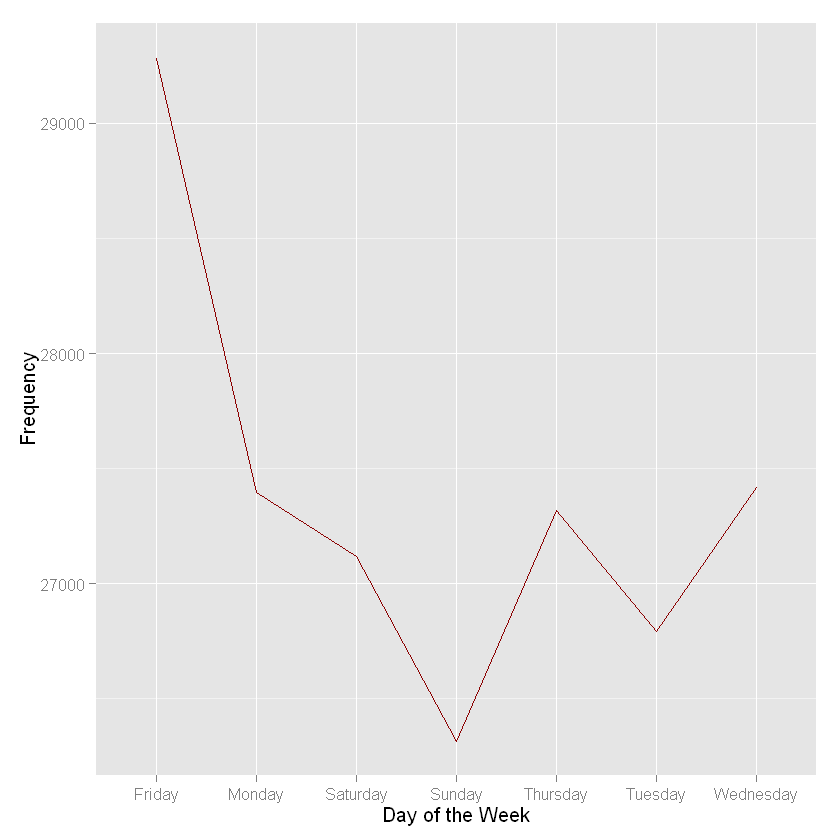The days of the week are out of order. Let's plot the days in cronological order

In :
DailyCrimeCounts$Var1 <- factor(DailyCrimeCounts$Var1, ordered=TRUE, levels=c("Monday", "Tuesday", "Wednesday", "Thursday", "Friday", "Saturday", "Sunday"))

In :
ggplot(DailyCrimeCounts, aes(x=Var1, y=Freq)) + geom_line(aes(group=1), color="darkred") + xlab("Day of the Week") + ylab("Total Motor Vehicle Thefts")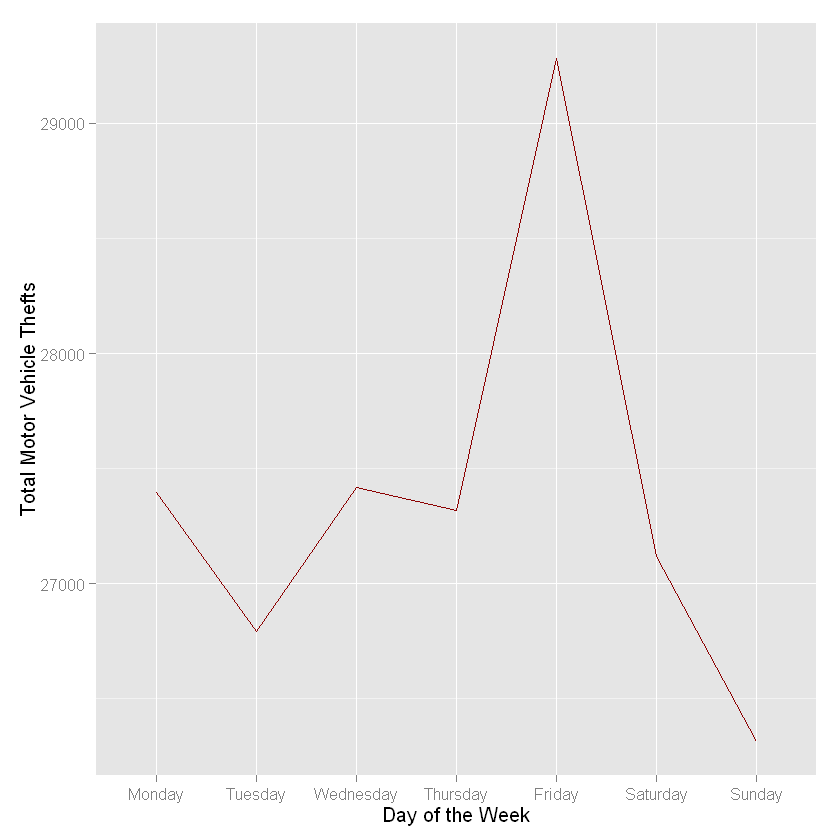Now let's add hour of the day and create a heatmap.

In :
table(mvt$Weekday, mvt$Hour)

Out:
               0    1    2    3    4    5    6    7    8    9   10   11   12
Friday    1873  932  743  560  473  602  839 1203 1268 1286  938  822 1207
Monday    1900  825  712  527  415  542  772 1123 1323 1235  971  737 1129
Saturday  2050 1267  985  836  652  508  541  650  858 1039  946  789 1204
Sunday    2028 1236 1019  838  607  461  478  483  615  864  884  787 1192
Thursday  1856  816  696  508  400  534  799 1135 1298 1301  932  731 1093
Tuesday   1691  777  603  464  414  520  845 1118 1175 1174  948  786 1108
Wednesday 1814  790  619  469  396  561  862 1140 1329 1237  947  763 1225

13   14   15   16   17   18   19   20   21   22   23
Friday     857  937 1140 1165 1318 1623 1652 1736 1881 2308 1921
Monday     824  958 1059 1136 1252 1518 1503 1622 1815 2009 1490
Saturday   767  963 1086 1055 1084 1348 1390 1570 1702 2078 1750
Sunday     789  959 1037 1083 1160 1389 1342 1706 1696 2079 1584
Thursday   752  831 1044 1131 1258 1510 1537 1668 1776 2134 1579
Tuesday    762  908 1071 1090 1274 1553 1496 1696 1816 2044 1458
Wednesday  804  863 1075 1076 1289 1580 1507 1718 1748 2093 1511
In :
HourlyTheftCounts <- as.data.frame(table(mvt$Weekday, mvt$Hour))
str(HourlyTheftCounts)

'data.frame':	168 obs. of  3 variables:
$Var1: Factor w/ 7 levels "Friday","Monday",..: 1 2 3 4 5 6 7 1 2 3 ...$ Var2: Factor w/ 24 levels "0","1","2","3",..: 1 1 1 1 1 1 1 2 2 2 ...
$Freq: int 1873 1900 2050 2028 1856 1691 1814 932 825 1267 ...  In : HourlyTheftCounts$Hour <- as.numeric(as.character(HourlyTheftCounts$Var2))  In : ggplot(HourlyTheftCounts, aes(x=Hour, y=Freq))+geom_line(aes(group=Var1, color=Var1), size=0)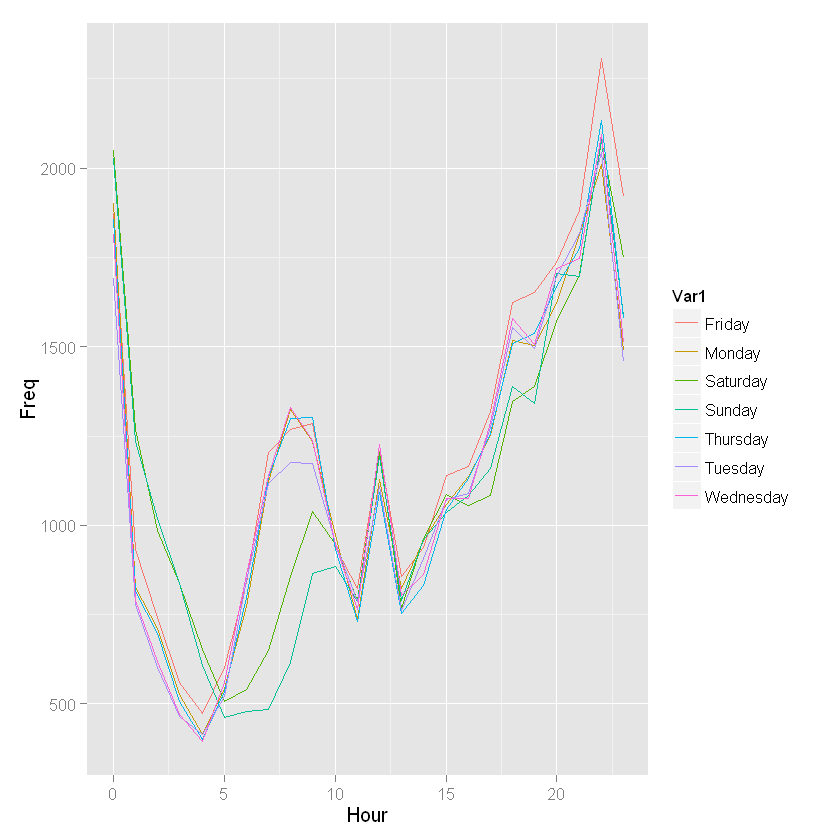This plot may not be easy to interpret looking at he sevel line at the same time. Let's create a heatmap and see if we can get a better visual representation. First, though, we need to order the weekdays in cronological order starting on Monday. In : HourlyTheftCounts$Var1 <- factor(HourlyTheftCounts\$Var1, ordered=TRUE, levels=c("Monday", "Tuesday", "Wednesday", "Thursday", "Friday", "Saturday", "Sunday"))

In :
# Now, create the heatmap
ggplot(HourlyTheftCounts, aes(x=Hour, y=Var1))+geom_tile(aes(fill=Freq)) + scale_fill_gradient(name="Total MV Thefts", low="white", high="red") + theme(axis.title.y=element_blank())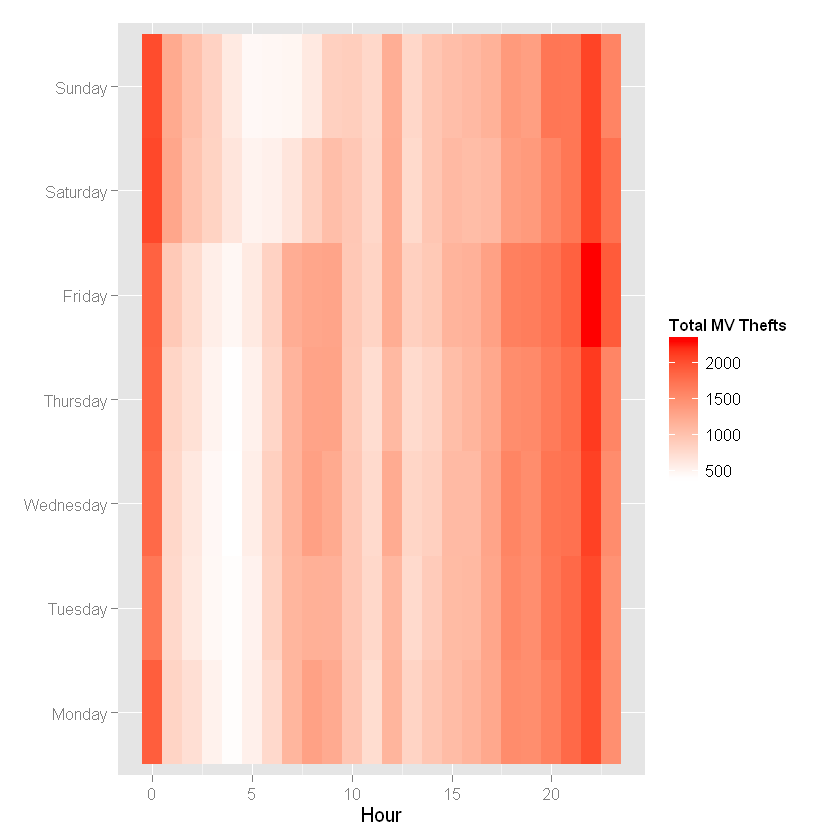In the following section, we will plot the heatmap for the theft rate on the map of Massachussetts.

In :
library(maps)

In :
install.packages("ggmap",repos='http://cran.us.r-project.org')

The downloaded binary packages are in C:\Users\Desta\AppData\Local\Temp\RtmpQFdndW\downloaded_packages
In :
library(ggmap)

In :
#4152252

#16# Load a map of Chicago into R:xxxccxc/

chicago <- get_map(location="Chicago", zoom=11)

Map from URL : http://maps.googleapis.com/maps/api/staticmap?center=Chicago&zoom=11&size=640x640&scale=2&maptype=terrain&language=en-EN&sensor=false

In :
#Look at the map
ggmap(chicago)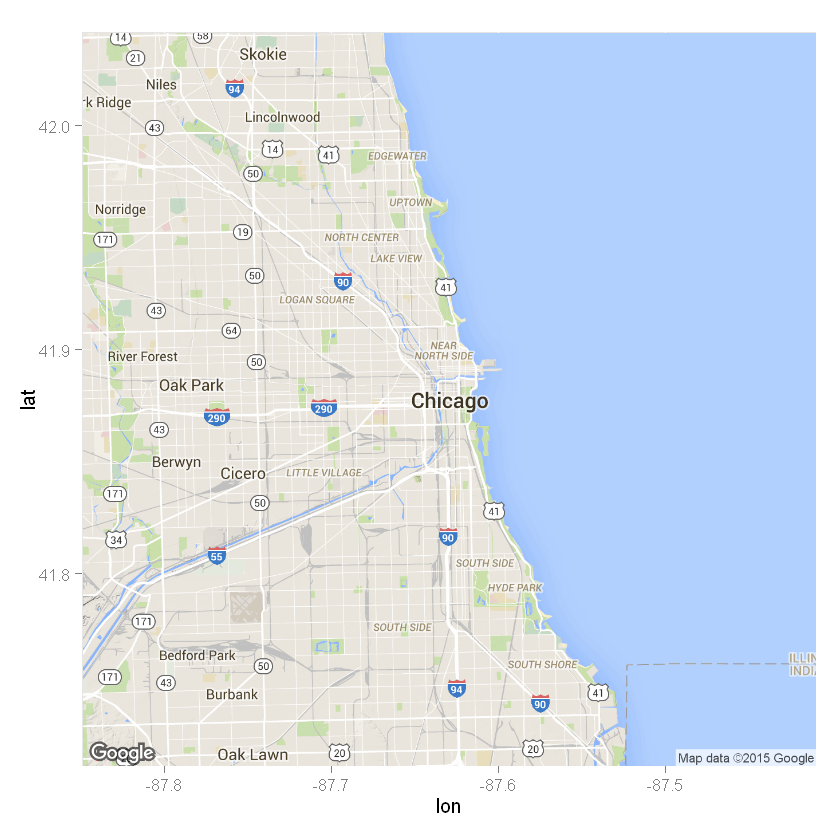In :
mvt <- read.csv("Data/mvt.csv", stringsAsFactors=FALSE)

In :
# Plot the first 100 motor vehicle thefts:
ggmap(chicago) + geom_point(data = mvt[1:100,], aes(x = Longitude, y = Latitude))